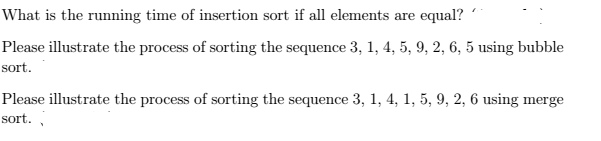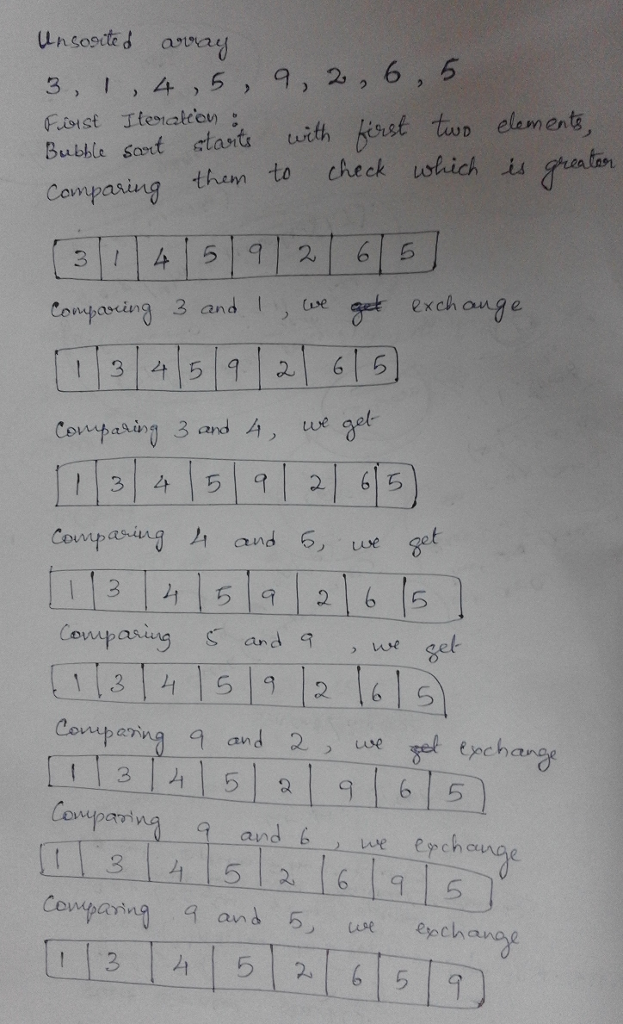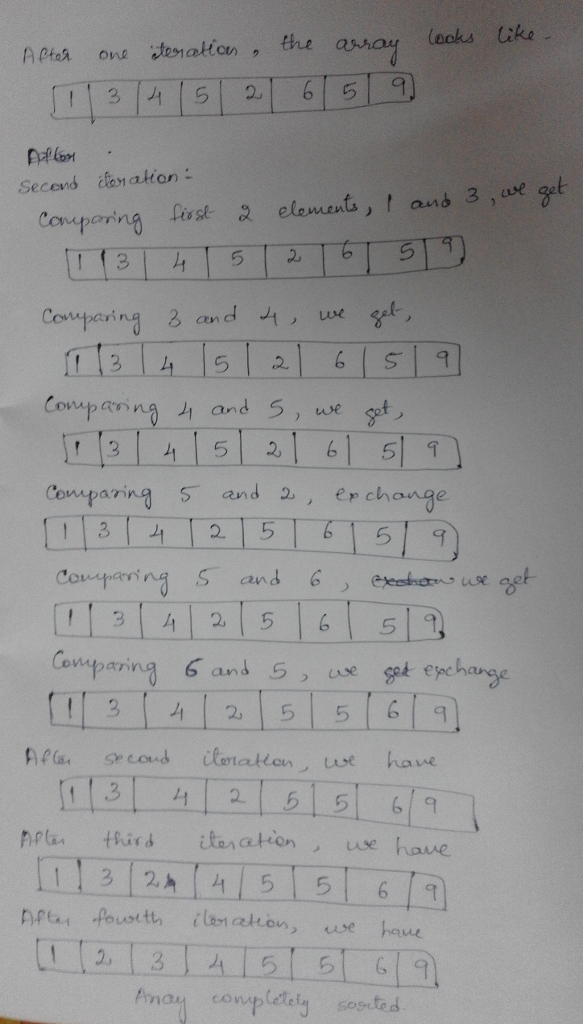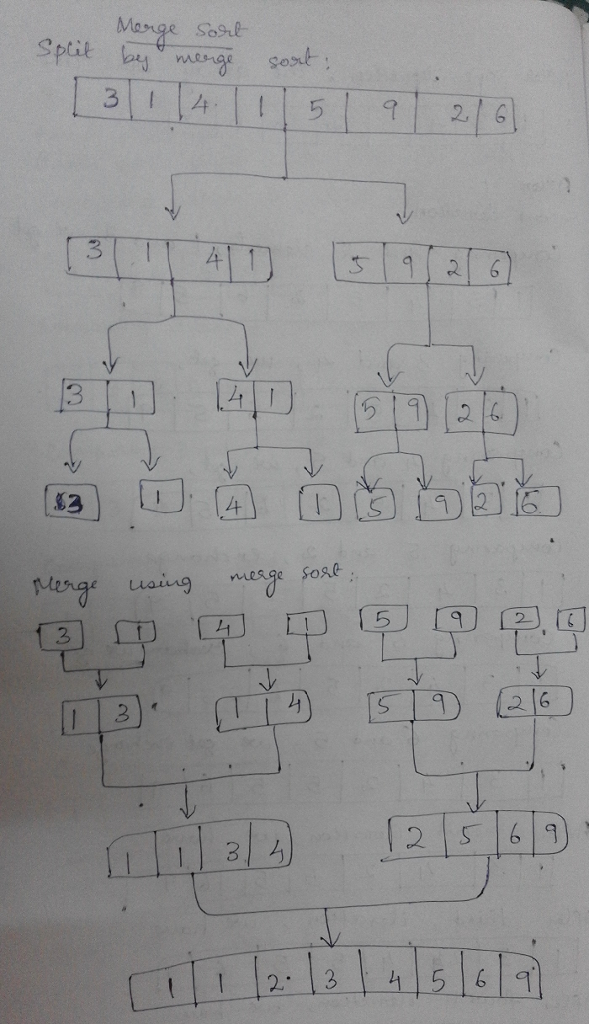# Answered! What is the running time of insertion sort if all elements are equal? Please illustrate the process of sorting the…What is the running time of insertion sort if all elements are equal? Please illustrate the process of sorting the sequence 3, 1, 4, 5, 9, 2, 6, 5 using bubble sort. Please illustrate the process of sorting the sequence 3, 1, 4, 1, 5, 9, 2, 6 using merge sort.

(1) The running time of insertion sort if all elements are equal : O(n)

Let A denote the array being sorted. For each element A[i], you check the preceding element A[i-1] and find they are equal. And you do not check further. Thus, you perform exactly n-1 comparisons, which makes the algorithm run in O(n) time.

Don't use plagiarized sources. Get Your Custom Essay on
Answered! What is the running time of insertion sort if all elements are equal? Please illustrate the process of sorting the…
GET AN ESSAY WRITTEN FOR YOU FROM AS LOW AS \$13/PAGE

(2) Bubble sort(3)